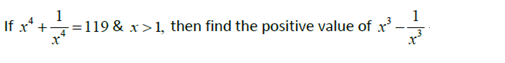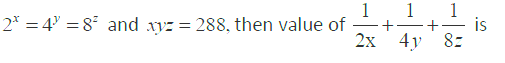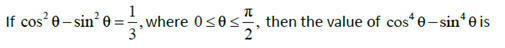Directions: (Q. 1 - 5): Solve the following questions -

1.(a) 25

(b) 27

(c) 36

(d) 49

2.(a) 11/12

(b) 11/96

(c) 29/96

(d) 27/96

3.(a) 1/3

(b) 2/3

(c) 1/9

(d) 2/9

4. If each angle of a triangle is less than the sum of the other two, then the triangle is

(a) obtuse angled

(b) right angled

(c) acute angled

(d) equilateral

5. If the measures of the sides of triangle are (x2 – 1), (x2 + 1) and 2x cm, then the triangle would be

(a) Equilateral

(b) acute-angled

(c) isosceles

(d) right-angled